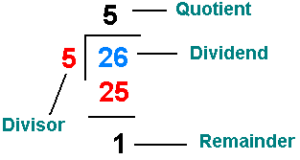# Java Program Calculate Remainder | Java programs

Java program to calculate the remainder program – So what exactly is a remainder? When a number is subtracted from another the remaining leftover is the remainder. Similarly when a number is divided from another, if there is a number left, it is called a remainder.

You bought a pen for rs 10 and gave the shopkeeper a 50 rs note. Now, that 40 rs leftover is the remainder. That’s it.

So the remainder can be rephrased as something that is leftover after a subtraction or a division. Let’s take a look at some examples to know how exactly the remainder works.

Example 1 – In the following image below, when 26 is divided with 5 it is left with a remainder of 5, as only numbers with “0” or “5” can be perfectly divisible by 5. Therefore, “1” is remaining in the given calculation.The remainder can be a Whole number like 4,10,13 etc or it can also be a number with a decimal like “2.4”, “3.5” etc. If 25 is divided by 5 the quotient is 5 and the remainder 0 which is a whole number but suppose if 25 is divided by 10 it will produce a quotient of 2 and a remainder of 5.

## Remainder Java Program

Output:

Explanation:

Java has a plethora of use cases and this blog is meant to demonstrate how to find the remainder in a Java program. We have a good amount of other Java programs too and incase if you are interested you can check those out here.  So let’s skip right into the program.

In this program, we’ll find the remainder of a division without making use of a modulus operator (%).

Before directly proceeding to the solution, we must always first see the problem statements, see for any constraints, figure out the inputs, decide the resultant expected output and then only jump into the logic or solution.

Here, the problem statement is to find the remainder without the use of a modulus operator. For this, we require two inputs i.e., the dividend and the divisor.

Since there is no mention of them to be the only integer, it could be float also so we make use of the double data type which also marks as our constraint. Our expected is a number representing the remainder when the divisor divides the dividend.

Now coming to the solution, we need to first get our inputs.

To make things convenient for the user, we read the input at runtime by making use of the Scanner class in Java.

This is used because, if we directly give values in the code itself then, for any change in the input one has to go to the code to make changes which is not very efficient way of writing a code.

Before reading input values, we first create an object for this Scanner class and then using this object, we call the nextDouble() method to read our inputs as follows:

After getting the input dividend (a) and divisor (b), we first initialize the remainder variable (r) to the dividend (a).

We keep subtracting the divisor (b) from the remainder(r) until we reach a state where remainder(r) is no longer greater than divisor(b). When it reaches this state, the value of remainder(r) is our resultant output.

for(r=a;r>b;r-=b);

A for loop, first initializes then, checks the condition. If the condition satisfies then, the statements inside the block is executed (in this case there are no statements). Then, the updating is done (r-=b or r=r-b) and again the condition is checked. This repeats until the condition is false.

Let us take an example to understand it better. Let our dividend a be 10 and our divisor b be 3.

1. First r=a //10
2. If  r>b  //10>3 then update is done
3. Then, r=r-b //r=10-3=7   Again condition is checked
4. If r>b  //10>7  True
5. Then, r-=b //r=7-3=4  Again condition is checked
6. If r>b  //4>3  True
7. Then,  r-=b  //r=4-3=1 Again condition is checked
8. If r>b  //1>3 False

So, it comes out of the loop and the resultant remainder(r) value is ‘1’. We all know that, even with modulus operator we will get the same value.

This way, without even making use of the Java  modulus operator(%), remainder can be found.

x

## Plus Star Pattern Java Program | Patterns

Java program to print plus star pattern program – We have written the below print/draw ...